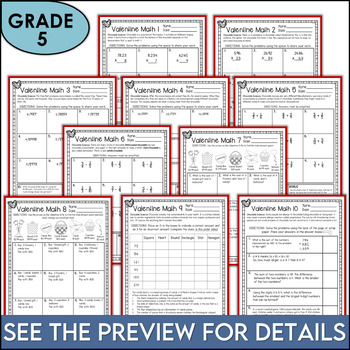# Valentine Math Print and Solve Gr. 5

Rated 4.93 out of 5, based on 14 reviews
14 Ratings;
4th - 5th
Subjects
Resource Type
Standards
Formats Included
• PDF
Pages
23 pages
Report this resource to TPT

### Description

Here's a great way to add some math to Valentine's Day events! These are quick practice sheet for math skills. My math sets were invented to fill a need that you might also have! This set of skill sheets has many more uses, too! Sub days are the perfect time to pull these out. Homework, math centers, early finishers, intervention, and more!

What makes this set unique? Each page includes science facts about chocolate! Win-win!

This set includes ten practice sheets covering these skills:

• Multiplication with decimal, 4 digits times 1 or 2 digits
• Multiplication with decimal, 3 or 4 digits times 2 digits
• Division with 1-digit divisor, 3-digit dividend, 3 or 4-digit quotient
• Division with 2-digit divisor, 3 or 4-digit dividend, 2 or 3-digit
• quotient
• Addition of fractions with unlike denominators
• Subtraction of fractions with unlike denominators
• Addition and Subtraction with money amounts
• Logic Puzzle
• Challenging Variety

The Worksheets:

• Pages include 10 problems and a bonus problem.
• The tenth problem and bonus problem may cover a different skill or multiple steps.
• The logic puzzle has clues to identify different shapes of candy boxes.
• The Multi-step page has five problems covering a variety of skills in a word-problem format.

Answer keys are included! The multistep page has an additional answer page showing how to solve the problems.

You might also enjoy these Valentine's Day Resources:

More Math Topics:

*****************************************************************************

Be the first to know about my new discounts, freebies, and product launches:

Thank you so much,

Teachers Really are Terrific!

Total Pages
23 pages
N/A
Teaching Duration
N/A
Report this resource to TPT
Reported resources will be reviewed by our team. Report this resource to let us know if this resource violates TPT’s content guidelines.

### Standards

to see state-specific standards (only available in the US).
Fluently multiply multi-digit whole numbers using the standard algorithm.
Find whole-number quotients of whole numbers with up to four-digit dividends and two-digit divisors, using strategies based on place value, the properties of operations, and/or the relationship between multiplication and division. Illustrate and explain the calculation by using equations, rectangular arrays, and/or area models.
Add and subtract fractions with unlike denominators (including mixed numbers) by replacing given fractions with equivalent fractions in such a way as to produce an equivalent sum or difference of fractions with like denominators. For example, 2/3 + 5/4 = 8/12 + 15/12 = 23/12. (In general, 𝘢/𝘣 + 𝘤/𝘥 = (𝘢𝘥 + 𝘣𝘤)/𝘣𝘥.)
Solve word problems involving addition and subtraction of fractions referring to the same whole, including cases of unlike denominators, e.g., by using visual fraction models or equations to represent the problem. Use benchmark fractions and number sense of fractions to estimate mentally and assess the reasonableness of answers. For example, recognize an incorrect result 2/5 + 1/2 = 3/7, by observing that 3/7 < 1/2.
Apply and extend previous understandings of multiplication to multiply a fraction or whole number by a fraction.